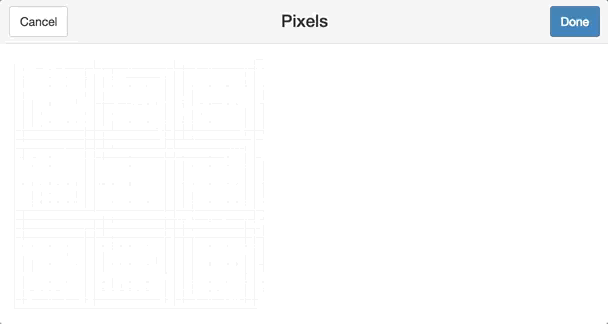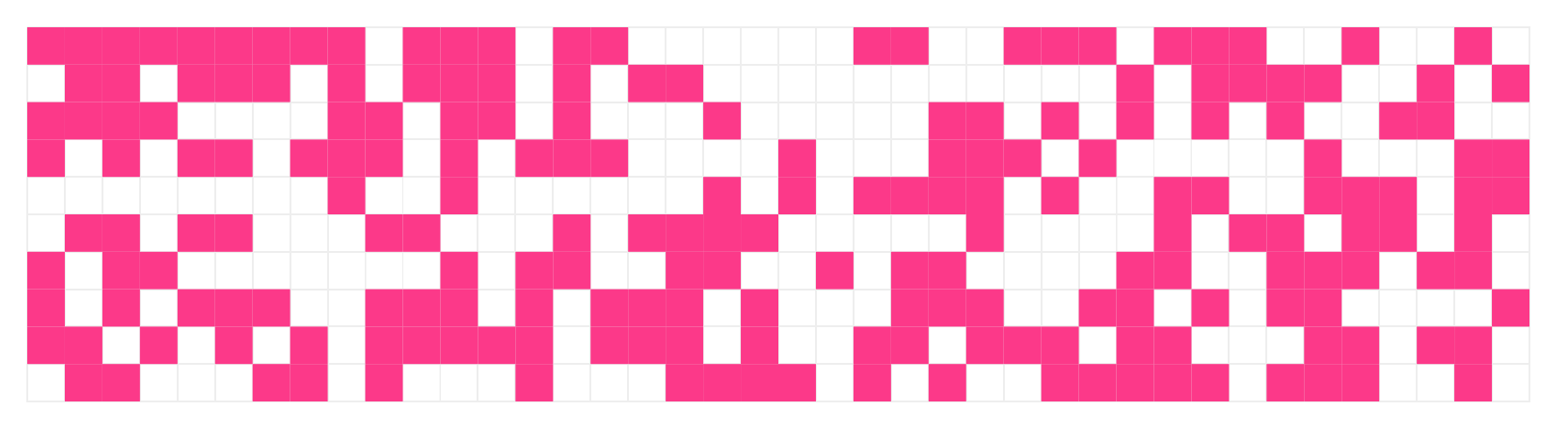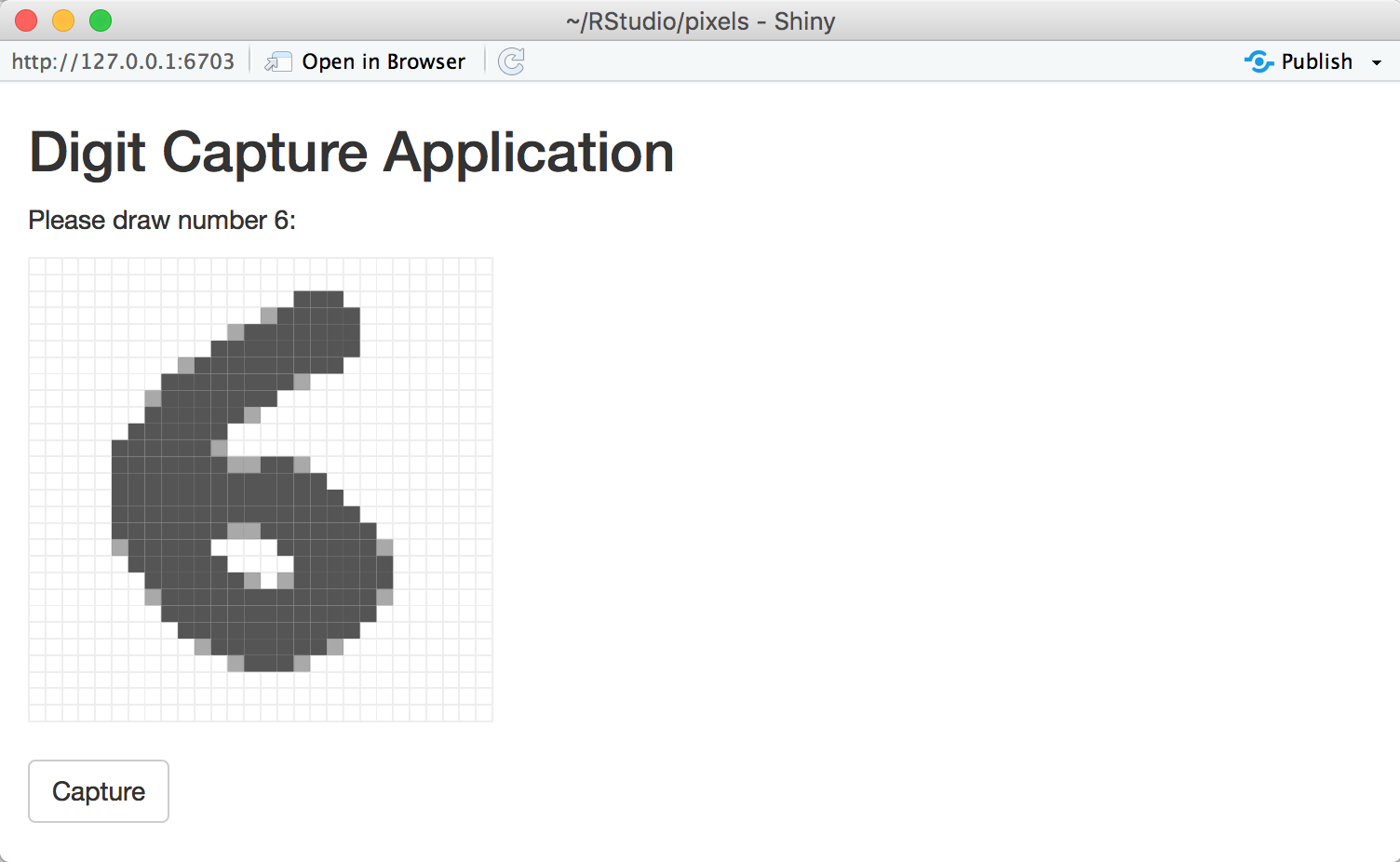# Tools for Working with Pixels in R

This package provides an htmlwidget and Shiny Gadget to render and draw pixels with ease.

To draw pixels run `get_pixels()` which will start the gadget to retrieve an array of numeric values representing each pixel in the image:

``````library(pixels)
get_pixels()``````To display pixels, use `show_pixels()` with a row-first vector as follows:

``````show_pixels(
round(runif(400, 0, 1)),
grid = c(40, 10),
size = c(800, 200),
params = list(fill = list(color = "#FF3388"))
)``````Finally, one can use ‘shiny_render_pixels()’ with a ‘Shiny’ application to help collect image datasets. For instance, to collect images similar to the MNIST dataset as follows:

``````library(shiny)

ui <- fluidPage(
tags\$style(HTML("
#pixels {
height: 270px !important;
margin-top: 10px;
}
"))
),
titlePanel("Digit Capture Application"),
textOutput("prompt"),
shiny_pixels_output("pixels"),
actionButton("captureDigit", "Capture")
)

server <- function(input, output) {
output\$pixels <- shiny_render_pixels(
show_pixels()
)

digit <- reactiveVal(floor(runif(1, 1, 10)))
output\$prompt <- renderText(paste0("Please draw number ", digit(), ":"))

observeEvent(input\$captureDigit, {
digit_path <- file.path("digits", digit())
if (!dir.exists(digit_path)) dir.create(digit_path, recursive = TRUE)
saveRDS(input\$pixels, paste0(digit_path, "/", as.numeric(Sys.time()), ".rds"))

digit(floor(runif(1, 1, 10)))
output\$pixels <- shiny_render_pixels(
show_pixels()
)
})
}

shinyApp(ui = ui, server = server)``````